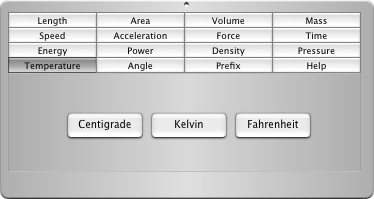RPN Scientific Calculator - Features - Conversion

# RPN Scientific Calculator - Features

Back to Features

## Units Conversion

RPN Scientific Calculator has the ability to easily convert physical quantities:
1. Type in or calculate a value
2. Press the units that correspond to the value entered
3. To calculate the value in another unit, press the desired unit
4. To calculate the value in yet another unit, press a different unit
5. Once a new value is typed in or calculated, the converter resets and is ready for step 2
6. If you typed in or calculated a new value and you want to recall the previous conversion, press recall (in memory drawer) and the desired units
7. If you typed in or calculated a new value and pressed the wrong original units, press store (in memory drawer) and then the correct units

### Complete List of Units Conversion

Length ConversionArea ConversionVolume ConversionMass ConversionSpeed ConversionAcceleration ConversionForce ConversionTime ConversionEnergy ConversionPower ConversionDensity ConversionPressure ConversionTemperature ConversionAngle ConversionPrefix ConversionHelp Conversion